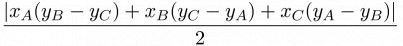### COCI 2007/2008, Contest #5

Ante bought a piece of land. The land contains N apple trees, but his piece is triangular and it is not easy for him to determine which apple trees belong to him.

Your program will be given the coordinates of the vertices of the triangle forming Ante's piece, and the coordinates of all apple trees. Determine the area of land belonging to Ante, and the number of trees belonging to him. We consider apple trees on the very border of his piece to belong to him.

The area of a triangle with vertices (xA, yA), (xB, yB) and (xC, yC) is given by the following formula:$\displaystyle \frac{|x_{A}(y_{B}-y_{C})+x_{B}(y_{C}-y_{A})+x_{C}(y_{A}-y_{B})|}{2}$

### Input

The first three lines contain the coordinates of the vertices of the triangle.

The following line contains the integer N (1 ≤ N ≤ 100), the number of apple trees.

Each of the following N lines contains the coordinates of one apple tree.

All coordinates are pairs of positive integers less than 1000, separated by a space.

### Output

Output the area of land belonging to Ante on the first line, with one digit after the decimal point.

Output the number of tree belonging to Ante on the second line.

### Input

1 1
5 1
3 3
4
3 1
3 2
3 3
3 4

### Output

4.0
3

### Input

3 2
5 4
1 6
3
2 4
3 5
4 3

### Output

6.0
3

### Input

2 6
5 1
7 8
5
1 4
3 5
6 4
6 5
4 7

### Output

15.5
2

Point Value: 7
Time Limit: 1.00s
Memory Limit: 32M